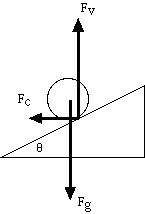Particle Accelerator

Small pushes delivered at just the right time can accelerate a coin rolling in a balloon to high speeds. In addition, as friction removes energy from the coin, it circles closer to the bottom of the balloon and slows down.

Material

• A spherical balloon 9 inches (25 cm) or more in diameter when inflated. Clearer balloons are better.
• A coin e.g. a quarter( optional, two coins.)

Assembly

Put the coin into the balloon.
Inflate the balloon.
Tie off the neck of the balloon.

To Do and Notice

Move the balloon in a horizontal circle.
Try to get the coin to roll in a circle around the inside of the balloon.

Notice that you can feel the coin as it passes over your hand.

Try to get the coin to go faster.
Notice that if you move your hands in a circle at just the right rate you can accelerate the coin to high speed.

As the coin speeds up it rolls higher in the balloon. At the highest speeds it orbits around inside the balloon at the equator.

Stop adding energy to the balloon. Notice that as the coin loses energy it moves more slowly and orbits closer to the bottom of the balloon.

What's Going On?

The coin rolls on its edge inside the bottom hemisphere of the balloon.

To stay in its orbit and not roll down the inclined plane to the bottom of the balloon an upward contact force from the balloon is needed. This contact force is the result of the motion of the coin in its circular orbit. The balloon must exert an inward centripetal force on the coin to accelerate it into its circular motion, at the same time the balloon exerts a vertical force on the coin.A ball rolling on an inclined plane: Gravity, Fg, pulls down, the contact force of the plane on the ball is resolved into two parts, a horizontal force, Fc, which provides the centripetal force and a vertical force, Fv. I have assumed there is no friction force so that the total contact force is perpendicular to the plane.

Math Root

A coin can be at rest on a horizontal surface.
However, as the angle of the inclined plane that the coin is rolling along increases, the upward force exerted by the inclined plane on the coin must increase to keep it from sliding down.

This upward force is the vertical part of the contact force of the inclined-plane wall of the balloon on the coin. If the coin is rolling along a banked curve, like the inside of a balloon, the horizontal component of the contact force must supply the centripetal force necessary to keep the coin moving in a circle.

The centripetal force to keep a coin of mass m moving at velocity v in a circle of radius r the required force is:

Fc = m v2/r

The vertical force necessary to keep a coin of mass m at rest while rolling along an inclined plane which rises at an angle q with respect to the horizontal is:

Fv = mg

Where g is the acceleration of gravity.

These forces are related by the requirement that their sum is perpendicular to the plane

Fc/Fv = tan q

so mv2/r = mg tan q
v2 = rg tan q

So that at lower angles the velocity is slower at higher angles the velocity is greater.

The balloon has lower angles near the bottom (and smaller radius too) so the velocity is slowest near the bottom.

So What?

A particle accelerator such as a cyclotron or a synchrotron pumps energy into an orbiting particle as it orbits inside the accelerator. The coin inside the balloon models the action of an accelerator.

Going Further

Optional Put two coins into a balloon, notice that if you start them orbiting in the same direction, they eventually roll around together.

Optional With two coins in the balloon it is possible to get the coins to orbit in opposite directions and to pump energy into both coins at the same time.

Get the coin orbiting inside the balloon. Then throw the balloon into the air. Notice that the balloon wobbles. A distant star wobbles when a planet orbits the star. From the wobble of the balloon we can tell there is a coin inside it, even if we cannot see the coin. The wobble of the star reveals the presence of an invisible planet.